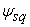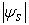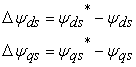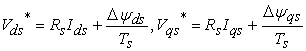﻿ Decoupled PWM Based Direct Torque Control of Four Level Inverter Fed Open End Winding Induction Motor Drive

### Decoupled PWM Based Direct Torque Control of Four Level Inverter Fed Open End Winding Induction Moto...

Satheesh G, T Bramhananda Reddy, CH Sai BabuOPEN ACCESSPEER-REVIEWED

## Decoupled PWM Based Direct Torque Control of Four Level Inverter Fed Open End Winding Induction Motor Drive

Satheesh G1,, T Bramhananda Reddy2, CH Sai Babu2

1Electrical and Electronics Engineering Department, G.Pulla Reddy Engineering College, Kurnool, India

2Electrical and Electronics Engineering Department, University college of Engineering Kakinada, JNTUK, Kakinada, India

### Abstract

A direct torque control (DTC) of open end winding induction motor (OEWIM) with decoupled PWM is proposed in this present paper. In this approach the four level voltages are generated by two conventional two level inverters which are fed with the unequal dc link voltages. These two inverters are feeding the induction motor from either ends. However, the proposed DTC scheme does not require the sector information of the estimated fundamental stator voltage vector and its relative position with respect to the stator flux vector. Simulation results clearly demonstrate a better dynamic and simplicity in numerical calculations of the proposed method.

### At a glance: Figures

• G, Satheesh, T Bramhananda Reddy, and CH Sai Babu. "Decoupled PWM Based Direct Torque Control of Four Level Inverter Fed Open End Winding Induction Motor Drive." Journal of Automation and Control 3.1 (2015): 18-24.
• G, S. , Reddy, T. B. , & Babu, C. S. (2015). Decoupled PWM Based Direct Torque Control of Four Level Inverter Fed Open End Winding Induction Motor Drive. Journal of Automation and Control, 3(1), 18-24.
• G, Satheesh, T Bramhananda Reddy, and CH Sai Babu. "Decoupled PWM Based Direct Torque Control of Four Level Inverter Fed Open End Winding Induction Motor Drive." Journal of Automation and Control 3, no. 1 (2015): 18-24.

 Import into BibTeX Import into EndNote Import into RefMan Import into RefWorks

### 1. Introduction

High power capability and multi level inverters controlling and simplicity in the controlling of the drive operation of the open end winding induction motor is attracting many researchers now a days. A high power configuration for the induction motor drive is proposed in  and later many researchers proposed many control configurations for the induction motor drives. Generation of three level voltages using the two conventional two- level inverters for open end winding induction motor is proposed in . After that many multi level configurations for open end winding induction motor are proposed in [4, 5, 6]. Two new approaches with decoupled strategy and Nearest Sub Hexagonal Centre strategies with the multi level output are proposed in . This configuration employed same amount of DC link voltage to feed the two two-level inverters. Another new Space Vector Pulse Width Modulation (SVPWM) is presented in  feeding the two two-level inverters with asymmetric DC link voltages. This method is the simple extension to the work proposed in .

A numerous direct torque controlling techniques are presented for the induction motor drives and a new approach with SVPWM based multi level inverter fed open end winding induction motor DTC control is presented in [13, 14, 15]. In  employed a decoupled strategy for the controlling of the open end winding induction motor.

In this paper, new DTC approach for the OEWIM with four level configuration is presented; this approach employs strategy specified in . Transient and steady state and step change in load simulation results are discussed.

### 2. OEWIM with Four Level Configuration

OEWIM drive with four level configuration is shown in Figure 1, where in each inverter is fed with an individual DC supply. If the DC-link voltages are equal, then three-level inversion is obtained . But in the proposed configuration, in order to generate four-level inversion Inverter-1 is having a DC voltage of (1/3)*Vdc, while inverter-2 is having DC voltage of (2/3)*Vdc.

Figure 1. The OEWIM configuration with 2 isolated unequal power supplies for four level configurations

The SV locations of the inverters 1 and 2 are depicted in Figure 2. In Table 1, it is shown that, how the four level are observed in line to line voltages with the specified configuration along with the pole voltages of individual inverters. The SV locations of the inverters 1 and 2 are depicted in Figure 2. In Table 1, it is shown that, how the four level are observed in line to line voltages with the specified configuration along with the pole voltages of individual inverters. As the inverters are two level inverters, then each inverter is having two values in the pole voltages. The inverter-1 outputs are either Vdc/3 or 0.

#### Table 1. Pole and Line voltages of the 4-level inverter

Similarly, inverter 2 outputs are either 2*Vdc/3 or 0. The difference of pole voltages of inverter 2 and 1 are shown in Table 1. The same can be visualized in top trace of the voltages in Figure 7. As there is no change in the configuration, except the magnitudes of the DC voltages, there is no path for the zero sequence currents.

### 3. Proposed DCPWM Algorithm for Four – Level OEWIM Configuration

A DCPWM algorithm, which is proposed in  for OEWIM configuration with three level output, is now used for the four-level output. In this PWM algorithm the reference vector Vref is constructed by subtracting the second voltage vector (-Vref/3) from the first voltage vector (2Vref/3). This method of PWM algorithm is shown in Figure 5.

Figure 4. Modulated waveforms for the individual inverters. Top trace modulated waveform for the first inverter, bottom trace modulated waveform for the second inverter with 180 degrees phase shift

With four-level OEWIM configuration the reference vector OT with a magnitude of |Vdc|∠α is generated which is shown in Figure 3. Now this reference vector is resolved into two vectors OA (|2Vdc/3 |∠α) and AT with (|Vdc/3 | ∠180° + α). However, in this approach, both inverters are to be switched.

The proposed algorithm for the four-level OEWIM drive is validated by using MATLAB simulation. The modulating signals for two inverters are depicted in Figure 4. These modulation signals are operated with 180 degrees phase shift. The generated pulses for two inverters are depicted in Figure 5.

### 4. DTC of Four-level OEWIM

The electromagnet torque of the open-end winding configuration of induction motor is calculated in a same manner as for normal induction motor using the standard torque equation as:Where P is the number of poles,&are the stator d-q axis flux and Isd & Isq are the d-q axis stator currents in the stationary reference frame. The schematic of the proposed method is as shown in Figure 8. In this method, speed of the reference stator flux vectoris derived by the addition of slip speed and actual rotor speed. The actual synchronous speed of the stator flux vectoris calculated from the adaptive motor model. After each sampling interval, actual stator flux vectoris corrected by the error and it tries to attain the reference flux space vector. Thus the flux error is minimized in each sampling interval. In this paper, the direct axis and qudrature axis components of the reference voltage vector are created by corresponding direct axis and quadrature axes stator flux error components respectively. They are derived as follows Reference value of the direct axis and quadrature axis stator fluxes and actual value of the direct axis and quadrature axis stator fluxes are compared in the reference voltage vector calculator block and hence the error in the direct and quadrature axes stator flux vectors is obtained asThe knowledge of flux error and stator resistive drop allows the determination of appropriate reference voltage space vectors along direct axis and quadrature axis, which is given asThe above derived direct & quadrature components of the reference voltage vector are then fed to the SVPWM block, from where the gating pulses for two inverters are generated.

### 5. Results and Discussion

Figure 10. Starting response in modulating waveform, phase and line voltages of DTC of DCPWM based four-level OEWIM drive
Figure 12. Steady state responses in modulating waveform and voltage waveforms of DTC of DCPWM based four-level OEWIM drive
Figure 14. Responses in modulating waveform, voltage waveforms of DTC of DCPWM based four-level OEWIM drive during load change

Several simulation studies are performed to validate the proposed DTC of four-level OEWIM drive. Different operative conditions like starting, steady state, change in load, reversal of speed etc. are observed. Figure 9 to Figure 16 shows the simulation results for DTC of DCPWM based four-level OEWIM drive.

To validate the proposed method, simulation studies have been carried out by using MATLAB /SIMULINK. The motor parameters are as follows 4 KW, 400V, 30 N-m, 1470 rpm, 4-pole, 50 Hz, 3-phase, stator resistance Rs = 1.57 ohm, rotor resistance Rr = 1.21 ohm, stator inductance Ls=0.17 H, rotor inductance Lr=0.17 H, mutual inductance Lm=0.165H, moment of inertia J=0.089 Kg-m2.

The starting response of the motor phase currents, speed, torque and flux are shown in Figure 9 in Figure 10 the modulating waveform, pole voltages of individual inverters and effective phase voltage is shown. The steady state operating period conditions and the responses of currents, torque, speed and voltage are depicted in Figure 11 and Figure 12 respectively.

Figure 16. Responses in modulating waveform, voltage waveforms of DTC of DCPWM based four-level OEWIM drive during speed reversal

Response of motor currents, torque, speed and stator flux during change in load which is instigated at 0.6 sec and released at 0.7 sec of DTC of DCPWM based four-level OEWIM drive is observed in Figure 13. With the application of load, the current increases and the torque value also increases with a small drop in the motor speed. It can be observed that, with the increment of both current and torque values the flux value is retained at 1 Wb. The pole voltages of the two inverters and effective motor phase voltage is shown in Figure 14.

The variations in the motor phase currents and voltages during the speed reversal from 1200 rpm to -1200 are shown in Figure 15 and Figure 16 respectively. Here a speed change is instigated at 0.8 sec.

5.1. Analysis of the Current and Torque

The THD is calculated for one cycle of the motor phase current. From the Figure 17, The fundamental harmonic magnitude is 100% and the harmonics at the half of the switching frequency, at the switching frequency and twice the switching frequency with sidebands can be viewed. It is observed that high peaks of the harmonics with sidebands can be viewed exactly at the switching frequency of the inverters.

Figure 18 and Figure 19 shows the electromagnetic torque responses of the OEWIM drive during the start-up and steady state respectively for the DTC of OEWIM drive. From the statistical analysis of the torque errors the distribution of the torque errors of start-up and steady state are listed in Table 2 and Table 3 respectively. The time range for statistics is from 0 to 0.1 sec and 0.45 to 0.55 sec for start-up and steady state respectively. There are totally 10,000 points.

Figure 19. Steady state torque responses of DTC of four level OEWIM drive based on DC-PWM algorithm

During the start-up of the induction motor, the proposed DC-PWM produced 43.76% torque error for greater than two. During steady state running period of the induction motor, the torque errors are distributed with magnitude of less than two only.

### 6. Conclusion

A simple, effective multi level configuration for the direct torque control of the open end winding induction motor drive with asymmetrical dc link voltages is presented in this paper. This proposed method is very simple and is extension of the DC-SVPWM three level OEWIM configurations. The described PWM scheme uses only the three instantaneous phase reference voltages for the implementation and do not require either sector identification or lookup tables. This strategy will greatly reduce the maintenance cost due to the advantages associated with the induction motor. Direct torque control strategy will improve the speed control capability and dynamic response. There are two inverters connected across both the ends of the stator winding. This has another advantage of controlling the induction motor by using only one inverter in the light load condition or in emergency.

### References

  Joohn-Sheok Kim and Seung-Ki Sul, “A novel voltage modulation technique of the space vector PWM”, in Conf. Rec. IPEC’95, Yokohama, Japan, 1995, pp. 742-747.In article  E. G. Shivakumar, K.Gopakumar, S.K. Sinha, Andre Pittet, V.T. Ranganathan, “Space Vector Control of Dual Inverter Fed Open-end Winding Induction Motor Drive”, EPE Journal, Vol. 12, No. 1, pp. 9-18 (2002).In article  H.Stemmler, P. Guggenbach, “Configurations of High-Power Voltage Source Inverter Drives”, Proc. EPE Conf., pp. 7-14, (1993).In article  M. R. Baiju, K. Gopakumar, K. K. Mohapatra, V. T.Somasekhar, L. Umanand, “Five-level inverter voltage space phasor generation for an open-end winding induction motor drive”, IEE Proc. on Electric Power Applications, Vol. 150, No. 5, pp. 531-538, (2003).In article  M. R. Baiju, K. Gopakumar, K.K. Mohapatra, V. T.Somasekhar L. Umanand, “A high resolution multilevel voltage space phasor generation for an open-end winding induction motor drive”, EPE Journal, Vol. 13, No. 4, pp. 29-37, (2003).In article  B. Venugopal Reddy, V.T.Somasekhar, Y. Kalyan, “Decoupled Space-Vector PWM strategies for a Four-Level Asymmetrical Open-End Winding Induction Motor Drive With waveform Symmetries” IEEE TRANS on INDUSTRIAL ELECTRONICS, VOL. 58, NO. 11, NOVEMBER 2011 pp. 5130-5141.In article  G.Satheesh, T. Bramhananda Reddy and Ch. SaiBabu, “Novel SVPWM Algorithm for Open end Winding Induction Motor Drive Using the Concept of Imaginary switching Times” IJAST, Vol. 2, No. 4, 2011, pp 44-92.In article  Arbind Kumar, B.G. Fernandes and K. Chatterjee, “Direct Torque Control of Open-end Winding Induction Motor Drive Using the Concept of Imaginary Switching Times for Marine Propulsion Systems”, IEEE, pp. 1504-1509.In article  S. Srinivas and V.T. Somasekhar, “Space-vector-based PWM switching strategies for a three-level dual-inverter-fed open-end winding induction motor drive and their comparative evaluation” IEEE Trans, IET Electr. Power Appl., 2008, 2, (1), pp. 19-31.In article  G. Buja, D. Casadei, and G. Serra, “Direct stator flux and torque control of an induction motor: theoretical analysis and experimental results,” in Proc. 24th Annu. Conf. IEEE Ind. Electron. Soc., Aachen, Germany, Sep. 31, 1998, vol. 1, pp. T50-T64.In article  J. Quan and J.Holtz, “Sensorless vector control of induction motors at very low speed using a nonlinear inverter model and parameter identification,” IEEE Trans. Ind. Appl., vol. 38, no. 4, pp. 1087-1095, Aug. 2002.In article  Arhind Kurnar, BG Fernandes, K Chatterjee. “Simplified Hybrid SVM Based Direct Torque Control of Thee Phase Induction Motor,” National conference on CClS 2W. Goa (India) Vol. l, pp 137-142. 2004.In article  Arbind Kumar, BG Fernandes, K Chatterjee, “Direct Torque Control of Three Phase Induction Motor Using SVPWM With-out Sector and Angle Determination”, EPE-PEMC 2004, Paper No. A-71121.In article  Arbind Kumar, BG Fernandes, K Chatterjee, “DTC of Open-End Winding Induction Motor Drive Using Space Vector Modulation With Reduced Switching Frequency,” IEEE-PESC, 2004, pp 1214-1219.In article  G.Satheesh, T. Bramhananda Reddy and Ch. Sai Babu, “DTC of Open End Winding Induction Motor fed by Two Space-Vector-Modulated Inverters”, IEEE-INDICON, 2011.In article  G.satheesh, T. Bramhananda reddy and CH. Sai babu. “Space-vector based pwm switching strategy for a four-level dual inverter fed open-end winding induction motor drive”, ICAESM-2012, India, pp: 111-115.In article